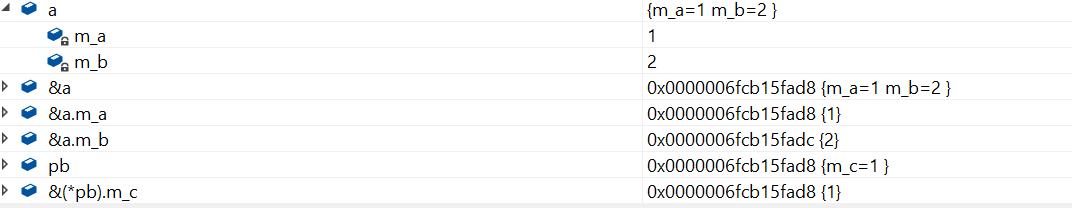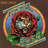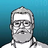V2EX = way to explore
V2EX 是一个关于分享和探索的地方

V2EX  ›  C++

# 我整理了这篇指针的知识点，想必对你有用

yiouejv · 57 天前 · 1151 次点击

### 指针和引用的区别

1. 非空区别: 任何情况下都不能使用指向空值的引用，一个引用必须总是指向某些对象。 指针可以指向空。
2. 合法性区别: 引用在使用之前不需要测试合法性，指针应该总是要被测试，防止其为空
3. 可修改性区别: 引用在初始化时指定对象，以后不能修改。

### 指针传递动态内存

``````#include<iostream>
#include<cstring>
using namespace std;

void getMemory(char*p, int num)
{
p = (char*)malloc(sizeof(char) * num);
}

int main(int argc, const char* argv[])
{
char *str = NULL;
getMemory(str, 100);
strcpy(str, "hello");
return 0;
}
``````

``````#include<iostream>
#include<cstring>
using namespace std;

void getMemory(char**p, int num)
{
*p = (char*)malloc(sizeof(char) * num);
}

int main(int argc, const char* argv[])
{
char *str = NULL;
getMemory(&str, 100);
strcpy(str, "hello");
cout << str << endl;
return 0;
}
``````

``````#include<iostream>
#include<cstring>
using namespace std;

char* getMemory(int num)
{
return (char*)malloc(sizeof(char) * num);
}

int main(int argc, const char* argv[])
{
char *str = NULL;
str = getMemory(100);
strcpy(str, "hello");
cout << str << endl;
return 0;
}
``````

``````char* strA()
{
char str[] = "hello world";
return str;
}
``````

str 里存的地址是函数 strA 栈帧里`"hello world"`的首地址，函数调用完成，临时空间被重置。

``````char* strA()
{
char *str = "hello world";
return str;
}
``````

`char str[]` 分配的是一个局部数组，

`char* str` 分配的是一个指针遍历，

`ISO C++ forbids converting a string constant to ‘char*’`

``````char* strA()
{
static char str[] = "hello world";
return str;
}
``````

``````#include<iostream>
using namespace std;

class A
{
public:
A() { m_a = 1; m_b = 2; }
~A(){}
void fun() { printf("%d%d", m_a, m_b);}
private:
int m_a;
int m_b;
};

class B
{
public:
B() {m_c = 3;}
~B();
void fun() {printf("%d", m_c);}
private:
int m_c;
};

int main(int argc, const char* argv[])
{
A a;
B *pb = (B*)(&a);
pb->fun();
return 0;
}
``````

`B* pb = (B*)(&a);`, 这是一个野蛮的转换，强制把 a 地址内容看成一个 B 类的对象，pb 指向的是 a 类的内存空间, 把 a 类空间按照 B 类的结构来解读。### 函数指针

``````#include<iostream>
using namespace std;

int Max(int x, int y)
{
return x > y ? x : y;
}

int main(int argc, char const *argv[])
{
int *p = &Max;
cout << p(2, 1) << endl;
return 0;
}
``````

`int *p` p 是 int 型的指针

`int *p(int, int)`, p 是一个函数，返回值为`int*`

`int (*p)(int, int)`, p 是一个指针，指向函数的地址，函数的返回值为 int

``````float(**def);
double*(*gh);
double(*f)();
int*((*b));
long(*fun)(int)
int(*(*F)(int, int))(int)
``````

``````float(**def);  // def 是二级指针，指向一级指针，一级指针指向数组，数组的大小为 10，数组元素类型为 float
double*(*gh);  // gh 是一级指针，指向一个数组，数组大小为 10，数组元素的类型为 double*
double(*f)();  // f 是一个数组，数组大小为 10，数组的元素类型为指针，指针指向的类型为 double() 的函数
int*((*b));  // b 是一个指针，指向一个数组，数组的大小为 10，数组元素的类型为 int*
long(*fun)(int)  // fun 是一个函数指针，指向 long(int) 型的函数
int(*(*F)(int, int))(int)  // F 是一个指针，指向一个函数，函数的参数为(int, int), 函数的返回值是一个指针，指向一个函数，函数的参数为(int), 函数的返回值为 int
``````

### 指针数组和数组指针

``````#include<iostream>
using namespace std;

int main(int argc, char const *argv[])
{
int v = {
{1,2,3,4,5,6,7,8,9,10},
{11,12,13,14,15,16,17,18,19,20},
};
int (*a) = v;       // 数组指针是一个二级指针
cout << a << endl;      // a 是一个指针，指向 {1,2,3,4,5,6,7,8,9,10}
cout << *a << endl;     // *a 也是一个指针，指向 1 的地址
cout << **a << endl;    // **a 取 1 的值

cout << a + 1 << endl;  // 指针向后偏移一个位置，这个位置的长度为指针所指容量的大小，偏移后指向 {11,12,13,14,15,16,17,18,19,20}
cout << *(a + 1) << endl;  // 和*a 的原理是一样的，指向 11 的地址
cout << **(a + 1) << endl;  // 取 11 的值

cout << *a + 1 << endl;  // *a 指向 1 的地址，*a + 1, 指针向后偏移一个位置，*a 指向的是 int 型的数据 m, 向后偏移 sizeof(int)，指向 2
cout << *(*a+1) << endl;  // 取 2
return 0;
}
``````

1. 一个整型数。
2. 一个指向整型数的指针。
3. 一个指向指针的指针，它指向的指针是一个指向一个整型数
4. 一个有 10 个整型数的数组
5. 一个有 10 个指针的数组，该指针是指向一个整型数的
6. 一个指向有 10 个整型数数组的指针
7. 一个指向函数的指针，该函数有一个整型参数并返回一个整型数
8. 一个有 10 个指针的数组，该指针指向一个函数，该函数有一个整型参数并返回一个整型数

``````// 1
int a;
// 2
int *a;
// 3
int **a;
// 4
int a;
// 5
int *a;
// 6
int (*a);
// 7
int (*a)(int);
// 8
int (*a)(int);
``````

``````int a[] = {1,2,3,4,5};
int *ptr = (int*)(&a + 1);
printf("%d %d", *(a+1), *(ptr - 1));
``````

``````#include<iostream>
#include<cstdio>
using namespace std;

int main(int argc, char const *argv[])
{
int a[] = {1,2,3,4,5};
int *ptr = (int*)(&a + 1);

cout << a << endl;  // 数组名的一个指向数组元素的常量指针
cout << &a << endl;  // &a 并不是一个指向常量指针的指针，而是一个指向整个数组的指针

// 以下两行验证以上的观点
cout << a + 1 << endl;
cout << &a + 1 << endl;

// 所以 a + 1 指向 2，*(a+1) 为 2
// &a + 1 应该指向 5 的下一个元素，ptr - 1 指向 5
printf("%d %d", *(a+1), *(ptr - 1));
return 0;
}
``````

### 迷途指针和野指针

``````#include<iostream>
using namespace std;

int main(int argc, char const *argv[])
{
char s1[] = "hello";
char s2[] = "the";
char s3[] = "world";
char* a[] = {s1, s2, s3};
char **pa = a;
pa++;
cout << *pa << endl;
return 0;
}
``````

a 是一个常量指针，指向数组的首地址，pa++, 向后挪一个指针大小，指向 s2, 输出 "the"

### this 指针

1. this 指针本质是一个函数参数，只是编译器隐藏起来的，语法层面的参数，实际上，成员函数默认第一个参数为 T* const this
2. this 在成员函数的开始前构造，结束后清除。

`a.func(10);` 会被编译器编译成 `A::func(&a, 10);`, 看起来和静态函数没区别，不过，区别还是有的。 编译器通常会对 this 指针做一些优化，this 指针的传递效率比较高，如 VC 通常是通过 ecx 寄存器传递。

1. this 指针并不占用对象空间，this 相当于非静态成员函数的一个隐含的参数，不占对象空间。
2. this 指针存放在何处？ this 指针会因编译器不同而有不同的位置，可能是堆，栈，也可能是寄存器。
3. this 指针是如何传递给类中的函数的？ 大多数编译器是通过 ecx 寄存器传递 this 指针，事实上，这也是一个潜规则，一半来说，不同编译器都会遵从一致的传参原则，否则不同的编译器产生的 obj 就无法匹配了
4. 我们只有获得一个对象后，才能通过对象使用 this 指针，如果我们知道对象 this 的位置，可以直接使用吗？

this 指针只有在成员函数中才有定义。 因此，你获得一个对象后，也不能通过对象使用 this 指针，只有在成员函数内才有 this4 条回复    2021-02-25 22:42:08 +08:001 wutiantong   57 天前7 所以中文编程技术社区一直存在的一个严重问题是，无论是在书本上还是论坛博客上，到处都可能充斥着似是而非，混淆概念，不够精确的表达。就以这篇文章来说：1. 指针可以指向空。2. 指针应该总是要被测试，防止其为空3. 编译器总是为函数的每个参数制作一个临时副本。4. p 申请了一个新的内存空间。。。并且 p 申请的内存。。。5. 我们可以直接把申请好的内存返回。6. str 里存的地址是函数 strA 栈帧里"hello world"的首地址7. （这里整段都是错误的，包括代码） char str[] 分配的是一个局部数组，char* str 分配的是一个指针遍历 。。。 指针变量是全局变量，它对应的是内存中的全局区域。8. （又在讲错误的东西）强制把 a 地址内容看成一个 B 类的对象，pb 指向的是 a 类的内存空间, 把 a 类空间按照 B 类的结构来解读。9. *a 也是一个指针，指向 1 的地址10. 数组名的一个指向数组元素的常量指针。。。&a 并不是一个指向常量指针的指针2 bfdh   56 天前 指针真的是越解释越乱，指针就是一个地址而已，那些所谓的指针类型不过是解读指针指向内容的方法而已，都不影响指针的本质。3 jones2000   56 天前 @bfdh "指针真的是越解释越乱" 这样才能有突出有难度,好忽悠, 1 句话就解释清楚了, 后面这么卖文章和课程呢.4 yiouejv   56 天前 我又不是培训机构，自己学习分享而已。不卖课哈

World is powered by solitude
VERSION: 3.9.8.5 · 25ms · UTC 18:03 · PVG 02:03 · LAX 11:03 · JFK 14:03
♥ Do have faith in what you're doing.